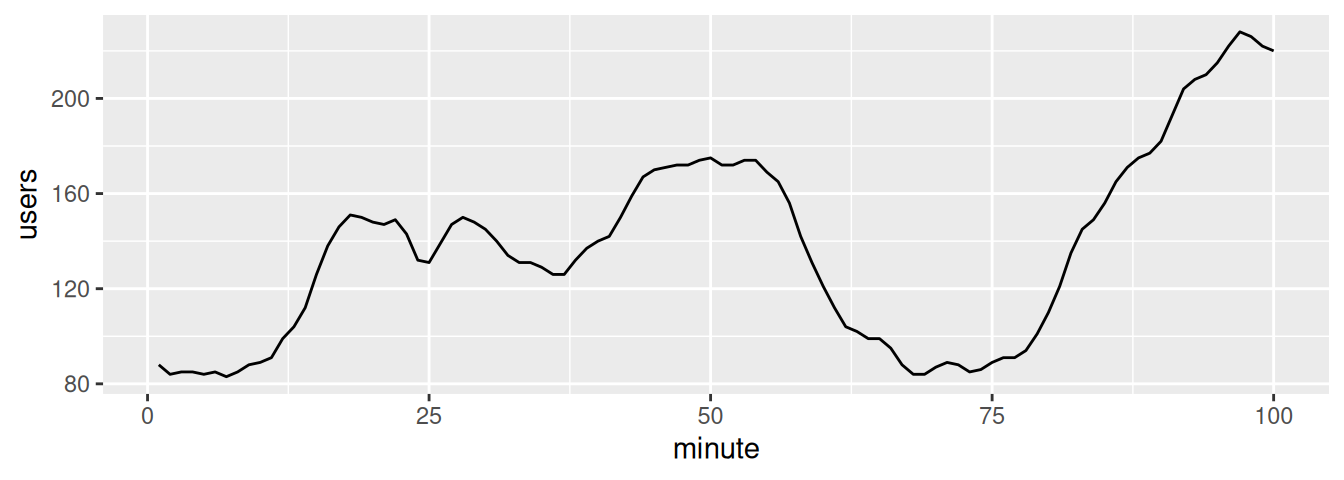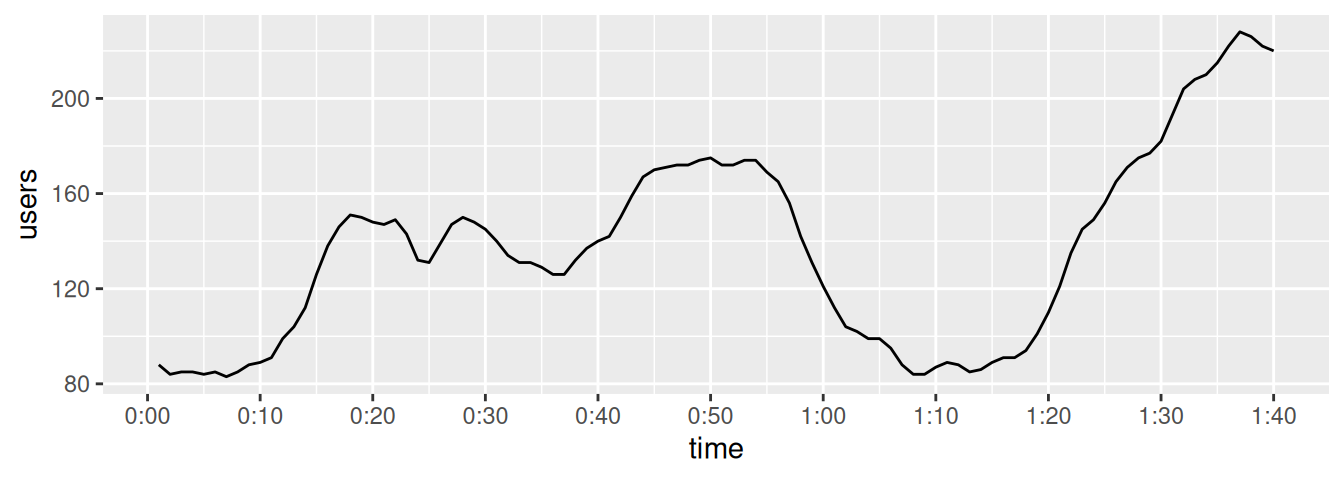## 8.18 Using Relative Times on an Axis

### 8.18.1 Problem

You want to use relative times on an axis.

### 8.18.2 Solution

Times are commonly stored as numbers. For example, the time of day can be stored as a number representing the hour. Time can also be stored as a number representing the number of minutes or seconds from some starting time. In these cases, you map a value to the x- or y-axis and use a formatter to generate the appropriate axis labels (Figure 8.40):

``````# Convert WWWusage time-series object to data frame
www <- data.frame(
minute = as.numeric(time(WWWusage)),
users  = as.numeric(WWWusage)
)

# Define a formatter function - converts time in minutes to a string
timeHM_formatter <- function(x) {
h <- floor(x/60)
m <- floor(x %% 60)
lab <- sprintf("%d:%02d", h, m) # Format the strings as HH:MM
return(lab)
}

# Default x axis
ggplot(www, aes(x = minute, y = users)) +
geom_line()

# With formatted times
ggplot(www, aes(x = minute, y = users)) +
geom_line() +
scale_x_continuous(
name = "time",
breaks = seq(0, 100, by = 10),
labels = timeHM_formatter
)``````Figure 8.40: Top: relative times on x-axis; bottom: with formatted times

### 8.18.3 Discussion

In some cases it might be simpler to specify the breaks and labels manually, with something like this:

``````scale_x_continuous(
breaks = c(0, 20, 40, 60, 80, 100),
labels = c("0:00", "0:20", "0:40", "1:00", "1:20", "1:40")
)``````

In the preceding example, we used the `timeHM_formatter()` function to convert the numeric time (in minutes) to a string like `"1:10"`:

``````timeHM_formatter(c(0, 50, 51, 59, 60, 130, 604))
#>  "0:00"  "0:50"  "0:51"  "0:59"  "1:00"  "2:10"  "10:04"``````

To convert to HH:MM:SS format, you can use the following formatter function:

``````timeHMS_formatter <- function(x) {
h <- floor(x/3600)
m <- floor((x/60) %% 60)
s <- round(x %% 60)                       # Round to nearest second
lab <- sprintf("%02d:%02d:%02d", h, m, s) # Format the strings as HH:MM:SS
lab <- sub("^00:", "", lab)               # Remove leading 00: if present
lab <- sub("^0", "", lab)                 # Remove leading 0 if present
return(lab)
}``````

Running it on some sample numbers yields:

``````timeHMS_formatter(c(20, 3000, 3075, 3559.2, 3600, 3606, 7813.8))
#>  "0:20"    "50:00"   "51:15"   "59:19"   "1:00:00" "1:00:06" "2:10:14"``````